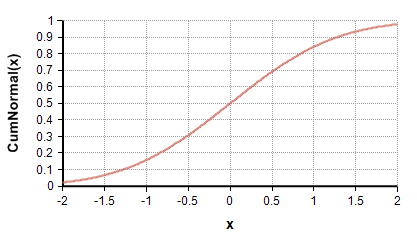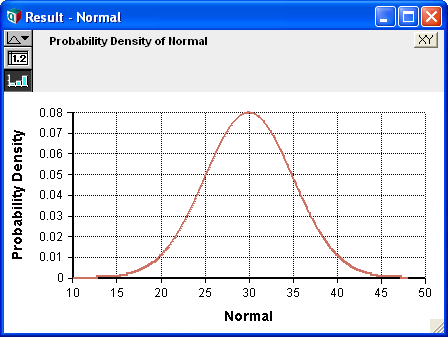# Normal distribution

(Redirected from CumNormal)

Release: 4.6  •  5.0  •  5.1  •  5.2  •  5.3  •  5.4  •  6.0  •  6.1  •  6.2  •  6.3  •  6.4

The normal distribution is a continuous, univariate, symmetric, unbounded, unimodal and bell-shaped probability distribution. It is a widely applicable distribution, specified by its mean and standard deviation. The central limit theorem states that the sum or average of a sufficiently long series of independent and identically distributed random numbers is approximately normally distributed when those numbers are sampled from any distribution with a finite mean and variance.

The range [mean - stddev, mean + stddev] encloses about 68% of the probability.

The multivariate generalization of the Normal distribution is the Gaussian distribution.## Functions

### Normal(mean, stddev, over)

The distribution function. Use this to describe a quantity that has a normal normal distribution with the given «mean» and standard deviation «stddev». The «stddev» must be 0 or greater.

As with all distribution functions in Analytica, Normal allows you to specify an optional parameter «over». Without this parameter, with scalar «mean» and «stddev» parameters, a single random normal variate is generated. If you want the random variate to vary independently across one or more indexes, then those indexes can be specified in «over». If the «mean» or «stddev» parameters are array-valued, then Normal returns an array of random values that are statistically independent across the indexes of the parameters.

### DensNormal(x, mean, stddev)

The probability density at «x», given by

$\displaystyle{ p(x) = {1\over{\sigma\sqrt{2\pi}}} e^{ - {1\over 2} \left( {{x-\mu}\over \sigma} \right)^2 } }$

### CumNormal(x, mean, stddev)

Returns the cumulative probability up to «x», given by

$\displaystyle{ p = Pr[x \le X] = {1\over{\sigma \sqrt{2\pi}}} \int_{-\infty}^x e^{- {1\over 2} {{(X-\mu)^2}\over\sigma^2} } dX = \frac12\left[1 + \mbox{erf}\left( \frac{x-\mu}{\sqrt{2\sigma^2}}\right)\right] }$

for a normal distribution with a mean $\displaystyle{ \mu }$ and standard deviation $\displaystyle{ \sigma }$. «Mean» and «stddev» are optional and default to Mean = 0, stddev = 1.

CumNormal(1) - CumNormal(-1) → .683

i.e., 68.3% of the area under a normal distribution is contained within one standard deviation of the mean.### CumNormalInv(p, mean, stddev)

The inverse cumulative density function, also called the quantile function. Returns the value x where CumNormal(x, mean, stddev) equals «p». The value that has a «p» probability of being greater than or equal to the true outcome.

## When to use

Use a normal distribution if the uncertain quantity is unimodal and symmetric and the upper and lower bounds are unknown, possibly very large or very small (unbounded). This distribution is particularly appropriate if you believe that the uncertain quantity is the sum or average of a large number of independent, random quantities.

## Statistics

The theoretical statistics (i.e., in the absence of sampling error) are

## Parameter Estimation

Suppose you want to fit a Normal distribution to historical data. Assume your data is in an array, x indexed by I. The parameters of the Normal distribution as obtained using:

«mean» := Mean(x, I)
«stddev» := SDeviation(x, I)

Central Limit Theorem
The CLT states that under non-degenerate conditions, the sum of a large number of independent random variables is approximately normally distributed. This holds even when the individual random variables are not normally distributed, and requires just that their distributions have finite variance. This property makes Normal distributions highly ubiquitous in statistics and in nature. Most common distributions end up being approximated by normal distributions in certain extremes.
Approximations by Normal Distribution
These are examples of distributions that are approximated by the Normal distribution:
• The Binomial(n, p) approaches Normal(n*p, n*p*(1 - p)) when n is large.
• The Poisson(mean) distribution approaches Normal(mean, Sqrt(mean)) as mean gets large.
• The ChiSquared(dof) distribution approaches Normal(dof, Sqrt(2*dof)) as dof gets large.
• The StudentT(dof) distribution approaches Normal(0, 1) when dof gets large.
• The Wilcoxon and Mann-Whitney-Wilcoxon tests (for whether two distributions are equal in hypothesis testing) use the so-called Wilcoxon distribution(s), which is/are quickly approximated by a Normal distribution.
Combination properties
• When $\displaystyle{ X \sim Normal(\mu,\sigma) }$, then
$\displaystyle{ a*X+b \sim Normal\left(a*\mu+b, a*\sigma\right) }$
• When $\displaystyle{ X_1 \sim Normal(\mu_1,\sigma_1) }$ and $\displaystyle{ X_2 \sim Normal(\mu_2,\sigma_2) }$, then
$\displaystyle{ a*X_1+b*X_2 \sim Normal\left(a*\mu_1+b*\mu_2, \sqrt{a^2 \sigma_1^2 + b^2 \sigma_2^2} \right) }$
• When a Bayesian Prior is normally distributed as $\displaystyle{ Normal(\mu,\sigma) }$, the posterior after observing a random value, x, drawn from the distribution, is also normally distributed as is given by
$\displaystyle{ Normal\left({{\mu+x}\over 2}, {\sigma\over\sqrt{2}}\right) }$
• The multivariate generalization of the Normal is the Gaussian distribution, parameterized by a mean vector an a Covariance matrix.

## Examples

Normal(30, 5) →### Brownian Motion

A discrete-time Brownian process in time can be encoded as:

Dynamic(0, Self[Time - 1] + Normal(0, 1))

or as

Cumulate(Normal(0, 1, over: Time), Time)

Please take note of several subtleties with these examples. First, these two are not quite equivalent -- they treat the @Time = 1 case differently. Either can be adjusted to treat the first time point as fixed or random - this is left as an exercise for the reader (solution is in the discussion tab). Second, notice that the «over» parameter was necessary in the Cumulate example. Without it, the expression:

Cumulate(Normal(0, 1), Time)

would select a single delta value that would apply to all time periods, resulting in a straight line with a random slope, rather than a random walk. The «over» parameter is not required in the Dynamic example since the recurrence expression is re-evaluated at each time step, causing the random variates to be independent automatically.